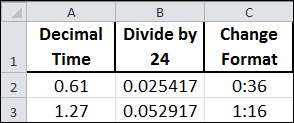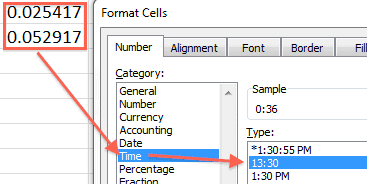# Convert Decimal Hours to Time in Excel

Converting a decimal time value to minutes and hours is easily done in Excel. That is, if the time value is less than 24 hours.## Decimal to Time Conversion

Here are three simple steps to convert decimal to time.

1. Enter a decimal value into a cell
2. Divide by 24
3. Change cell formatting to Time (h:mm)

To change the cell format use keyboard shortcut Ctrl+1 to bring up the Format Cells dialog box, select the Number tab, click Time, then select the h:ss format as shown below.If  you have a lot of conversions then one column for the decimal values and another column for a formula dividing by 24 will suffice. Just change the formatting for the second column. The example above shows three columns for illustrative purposes and is not very practical.

Obviously a conversion can be done in one cell, if you:

• Enter a formula into a cell with a decimal value divided by 24 (=0.61/24)
• Format that cell to h:mm

And remember, this only works properly for decimal values less than 24 hours.## 31 thoughts on “Convert Decimal Hours to Time in Excel”

1.Jiri

How can I convert decimal value bigger than 24 hours? For example, how do I convert 45.5 hours into format 45:30 ???

1.Gregory

That’s always a hard one. I had to look it up again here.

Essentially you divide the number (45.5) by 24, then change the cell formatting to a custom format [h]:mm and Excel will do the work for you. (Putting the h inside brackets means the time will be cumulative.)

1.Jiri

Thank you very much indeed! I could not find this detail (the function of the brackets) anywhere.

2.Luis Serpa

Hello,

I am trying to find a solution for a problem with time calculation.

I want to find how many days and hours will a trip last at different speeds (and then add this value to a starting date to otain an ETA, but this is easy).

Until now I simply divided the distance by the speed and then by 24, but this works only to distances/speed that led to trip duration inferior to one month.

With duration superior to that value the formula only returns values that exceed the 30 days.

You can hav an example by dividing 10’000 miles by a speed of 5 knots. Result is 200o hours which is 83,3 days. If I fromat that in a dd”d” hh”h” format it returns 23d 08h.

Maybe the problem is only the formatting, but I would like to be able to obtain 83d 08h.

How can i do that?

Luis Serpa

1.Gregory

=INT(2000/24)&”d “&TEXT((2000/24)-INT(2000/24),”h”) & “h”

and give you 83d 8h.

1.Luis Serpa

Many thanks.

1.Luís Serpa

Sorry, only now did I try your solution, but it does not work: the result is text, and it is not possible to do any calculation with it.

I’m trying with IF function (IF value > 30 then…) but it seems too heavy a solution. There should be a simpler way.

Thanks
Luis

3.Dawn Cunningham

Start time is 07:30
End time is 16:00
Lunch hour is .5

I need the forumla using these time formats for how many hours worked.

So far, I have this: =TEXT(B2-A2,”h:mm”)
Which is working… until I tried to put in the minus .5 for lunch.

Thanks so much!

1.Gregory

Assume cell A2 = 7:30 and cell B2 = 16:00 then lunch time should be in the same unit of measure. Instead of using decimal hours for lunch, 0.5 hours, you should use the same time format 0:30. Assume that in cell C2 you enter 0:30, then a formula in cell D2 would be =B2-A2-C2 and would result in 8:00. End time minus start time minus lunch duration (in time format h:mm) equals hours worked.

1.Dawn Cunningham

Hi Gregory…

I was not “allowed” to change the .5 and they have kept their formula “hidden” or “locked” somehow. When I did try the :30 it wasn’t working anyways.

HOWEVER, when I added the “0” in front bazinga! it works! 😀

Thank you very much for your help! And “they” will just have to deal with the change! LOL

1.Dawn Cunningham

Oh, Ok, so what happens when I use this formula my calculation of hours is kept in the time format. So, 8.5 hours shows up as 8:30 and that is not what I am required to have.
I am trying to format those specific cells to show the 8.5 but I am not figuring it out yet.
If you have anything further to suggest that would be great!!! 🙂
Just to recap, I need to calculate the amount of hours:

A1=Start Time 07:30
B1=End Time 16:00
C1=Lunch is .5 (half an hour)
D1=Number of hours worked is: ??.??

1.Gregory

D1 = (B1-A1)*24-C1

2.Dawn Cunningham

THANK YOU !!!

WOW, you have just saved me SO much time!

4.Marcus Stavchasky

Random question,

Can one set up a cell to receive 4 digits and have those digits convert to the hh:mm format after pressing enter?

For example, I enter in 1635 and the cell reads 16:35, Or I enter in 0957 and the cell reads 09:57

-Stav

1.Gregory

It is possible if you write some VBA code to handle the complexities.

I had another reader leave a comment about entering 3 or 4 digits for military time, leaving out the delimiter (:) and calculating the difference between End and Start times. I sent him a spreadsheet with all the calculations that you have to go through to make this work. It’s not difficult, just detailed.

So advancing that idea one more level by having VBA recognize input for cells in a particular column, and then making a transformation to regular time format is not that difficult. Just detailed and involved.

You’ve given me an idea for a couple of blog posts. Thanks.

5.Marcus Stavchansky

Do you mind linking me to your blog if you plan to share a solution using VBA code. I am very interested in learning a solution for my issue.

1.Gregory

You can subscribe to my blog on the main page. When I write this post, I’ll email you the link.

6.Jamal Rabbani

I would like to conver 2:31 (2 hrs 31 minutes) into minutes and then divide by number of calls to find average time per call. How do i convert number to minutes?

1.Gregory

Assume that 2:31 is in cell A1 and the number of calls is in cell B1, which the value is 10 for this example. The formula you want is:

=(A1*24*60)/B1

and make sure you cell formatting is General. The formula returns 15.1 minutes per call.

The 2:31 is multiplied by 24 hours/day and 60 minutes/hour to convert to 151 minutes. You can also use 1440, which is the number of minutes in a day (24 X 60). Then you simply divide by number of calls to get minutes per call.

7.Adolfo

Guys,

I used your procedure for Calculating Hours Between 2 Dates and Times… easy… Now I’m using your Convert Decimal Hours to Time in Excel, but I have some ranges with more than 24 hours, so as you mentioned, it doesn’t works properly… there is a way to make it work with more than 24 hours?

1.Gregory

Change your cell formatting to add a square bracket [] around the h in the time format. Here’s how: In the Format Cells dialog box (CTRL+1 on a PC and CMD+1 on a Mac) click the Number tab, in the Category box select Custom, then in the Type: box enter [h]:mm and the hour will accumulate past 24 hours.

1.adolfo

You are the man Greg… works perfectly.. THX!!!

8.Deb

Hi Gregory,

I am learning Excel and need help on calculating the total time (hours and minutes) worked in a day.
For example: Start time was 8:50am, End time was 3:28pm

Thank you so much,
Deb

1.Gregory

Assume 8:50 AM is in cell A2 and 3:28 PM is in cell B2. If you put the calculation =B2-A2 into cell C2, the only thing left is to select cell C2 and bring up the Format Cells dialog box (keyboard shortcut CTRL+1 on a PC and CMD+1 on a Mac) then click the Number tab, and in the Category box select Time, and finally choose a format like 13:30 which has no AM or PM associated with it. The result will be 6:38 and is the result you are looking for.

9.Borune RoyChoudhury

Hi Gregory,

Unfortunetly when working on Excel I put time using decimals. Eg. 17.25 was actually meant to be 17 hours and 25 minutes. Now when I try to convert that into the hh:mm:ss format in excel I’m getting 17:15:00 instead of 17:25:00.
Is there a way to read the decimal in excel as the minute value only?

Thanks.

1.Borune RoyChoudhury

Hi Gregory,

I kept looking for the answer and I Got It… it is =DOLLARDE(A1,60)/24 for the value in decimal in A1 using the cell format [h]:mm:ss.

Thanks anyway 🙂

1.Gregory

Don’t know about the formula you found, but his will work as well.

=TIME(INT(A1),MOD(A1,1)*60,0)

Cheers.

10.Bal

I’ve read everything here and can’t find an answer for my problem. I have created a format (00\:00) to enter my time in a cell. This allows me to simply enter a number without having to enter the colon. My problem is I can’t figure how to calculate this as time. Example: 0945-1800=8:15 but it calculates out as 8:55 because it is simply subtracting. Can you tell me, how I can make it calculate as hours.

1.Gregory

Assume 0945 is in cell A1 and 1800 is in cell B1. You have to divide by 100 and then get the integer and fractional part. In cell A2 enter the formula:

=INT(A1/100)+MOD(A1/100,1)*100/60 which will return 9.75

In cell B2 enter the formula =INT(B1/100)+MOD(B1/100,1)*100/60 which will give you 18.

And finally in cell C2 enter the formula:

=(INT(B2-A2)+(MOD(B2-A2,1)*60)/100)*100 to convert back to your original format, which returns 815 as the answer.

11.Gem

Hi! I need help badly. How can I have 13:15 PM in excel?It doesn’t have a PM on it.. Just 13:15

1.Gregory

You either get 13:15 which is military time and doesn’t have PM or you have 1:15 PM. Both formats are controlled in the Format Cells dialog box. Use the keyboard shortcut Control+1 on a PC or Command+1 on a Mac to bring up the dialog box. Then select the Number tab, and in the Category box select Time. Then in the Type box pick the format that best suits your needs and click OK.